• 内容索引:VC/C++源码,算法相关,离散数学,真值表　不用介绍了吧，离散数学的玩意，求真值表，用C++编程序实现的，可以学习一下哦。
• 大一菜鸡肝了近两个小时的成果，用于计算真值表;拿来水一篇博客(并不);代码中比较重要的两部分是原式向后缀式的转换，遍历所有原子命题的可能取值;具体的细节看代码吧，尽量添加了注释;#includeusing namespace std;...

大一菜鸡肝了近两个小时的成果，用于计算真值表;

拿来水一篇博客(并不);

代码中比较重要的两部分是原式向后缀式的转换，遍历所有原子命题的可能取值;

具体的细节看代码吧，尽量添加了注释;

#include

using namespace std;

const int maxn = 105;

const int maxstr = 1e5 + 10;

char s[maxstr],str[maxstr],Vstr[maxn];//依次为原式，后缀式，存放原子命题的符号

bool var[maxn];//不同原子命题的真值

mapv;//储存原子命题对应的编号

void Print_true(bool b){//打印bool对应的真值符号

if(b) printf("T\t");

else printf("F\t");

}

int Priority(char c){//返回运算符的优先级

int p;

switch (c) {

case '!': p = 5; break;

case '&': p = 4; break;

case '|': p = 3; break;

case '-': p = 2; break;

case '=': p = 1; break;

default : p = 0; break;

}

return p;

}

bool ToPostfix(){//原式转化为后缀表达式

int cnt = 0;

int len = strlen(s);

stackope;

for(int i=0; i

if(s[i] == '('){

ope.push(s[i]);

}

else if(s[i] == ')'){

if(ope.empty()) return false;

while(ope.top() != '('){

str[cnt++] = ope.top();

ope.pop();

if(ope.empty()) return false;

}

ope.pop();

}

else if(Priority(s[i]) == 0){

str[cnt++] = s[i];

}

else {

if(ope.empty()){

ope.push(s[i]);

}

else {

if(Priority(s[i]) > Priority(ope.top())){

ope.push(s[i]);

}

else{

while(!ope.empty() && ope.top() != '(' && Priority(s[i]) <= Priority(ope.top())) {

str[cnt++] = ope.top();

ope.pop();

}

ope.push(s[i]);

}

}

}

}

while(!ope.empty()){

str[cnt++] = ope.top();

ope.pop();

}

str[cnt] = 0;

return true;

}

bool Calculate(bool a, bool b, char ope){//进行真值的运算

bool ans;

if(ope == '&'){

if(a == true && b == true) ans = true;

else ans = false;

}

else if(ope == '|'){

if(a == true || b == true) ans = true;

else ans = false;

}

else if(ope == '-'){

if(a == true && b == false) ans = false;

else ans = true;

}

else if(ope == '='){

if(a == b) ans = true;

else ans = false;

}

return ans;

}

void init_var(int n, int sum){//对var数组初始化

while(sum>0){

bool x = sum%2;

var[n--] = x;

sum /= 2;

}

do{

var[n--] = false;

}while(n > 0);

}

bool Result(){//对后缀式进行计算

stackres;

int len = strlen(str);

for(int i=0; i

if(str[i] == '!'){

if(res.empty()){

printf("计算出现异常!\n");

}

else {

bool f = res.top();

// printf("text = %d\n",f);

res.pop();

res.push(!f);

}

}

else if(Priority(str[i])){

bool a,b;

if(res.empty()) printf("计算出现异常!\n");

else {

b = res.top();

res.pop();

}

if(res.empty()) printf("计算出现异常!\n");

else {

a = res.top();

res.pop();

}

bool ans = Calculate(a, b, str[i]);

res.push(ans);

}

else {

res.push(var[v[str[i]]]);

}

}

return res.top();

}

void Print_Out(){//打印提示语

printf("您好，欢迎使用离散数学真值表计算V1.2版本。\n");

printf("使用说明：数据为多组输入，每组一行，直接输入命题公式即可。\n");

printf("注：命题变元必须使用小写或大写字母表示。\n");

printf("联结词说明(英文符号): 非:! 合取:& 析取:|(逻辑或) 条件:-(负号) 双条件:=\n");

}

void NumOfVar(int &n){//提取命题公式中的变元

int len = strlen(s);

bool asi;

memset(asi, false, sizeof(asi));

for(int i=0; i

asi[s[i]] = true;

}

n = 0;

for(int i='A'; i<='Z'; i++){

if(asi[i]){

Vstr[++n] = i;

v[i] = n;

}

}

for(int i='a'; i<='z'; i++){

if(asi[i]){

Vstr[++n] = i;

v[i] = n;

}

}

}

int main(){

int n,cnt=0;

Print_Out();

printf("\n请输入第%d组：\n",++cnt);

while(~scanf("%s",s)){

v.clear();

NumOfVar(n);

if(ToPostfix()==false){

printf("您输入的原式错误\n");

}

else {

//标题栏的输出

for(int i=1; i<=n; i++){

printf("%c\t",Vstr[i]);

}

printf("%s\n",s);

//打印各部分真值

int m = pow(2,n);

for(int i=0; i

init_var(n, i);

for(int j=1; j<=n; j++){

Print_true(var[j]);

}

bool ans = Result();

Print_true(ans);

printf("\n");

}

printf("\n");

printf("请输入第%d组：\n",++cnt);

}

}

return 0;

}

展开全文• 以 ~ ^ & > - 分别表示 非 析取 合取 条件 双条件 连接词 以单个大写字母表示变量（支持26个变量） 以字符0或者1表示，式子中的T与F，支持 ( )（括号），有界面，下载后可直接运行
• 公式是由命题变元，逻辑连接词，括号组成的合法符号串，而命题变元是一个抽象概念
• 1、真值表：把变量的各种可能取值与想对应的函数值，用表格的形式一一列举出来，这种表格就叫做真值表 2、命题公式的析（合）取范式 ⑴文字：命题变元及其否定统称为文字 ⑵简单析取式：仅有限个文字组成的析取式 ⑶...离散数学
• 离散数学真值表（c语言编程实现）

万次阅读 多人点赞 2018-10-14 13:08:21
代码如下： 废话不多说： 主要利用二进制的转化实现  #include &lt;iostream&gt; #include &lt;math.h&gt; using namespace std; void shuru(char *p,int s); void shuchu(char *p,int s);...v...

代码如下：

废话不多说：

主要利用二进制的转化实现

#include <iostream>
#include <math.h>
using namespace std;
void shuru(char *p,int s);
void shuchu(char *p,int s);
void panduan(int s,int p,char *a,char b);
void shizi(char *p,int s);
void shiz(char *p,int s,char *a,char b);
char b={'p','q','r','s','d','m','n','l','j','k'};
int main()
{
int n;
cout<<"input the value of n"<<endl;
while(!(cin>>n))
{
cin.clear();
cin.sync();
cout << "不是数字"<<endl;
}
cout<<"input T or F"<<endl;
char a;
shuru(a,n);
cout<<"----------------------"<<endl;
for(int i=0;i<n;i++){//根据命题数输出命题符号
cout<<b[i]<<"		";
}
cout<<"VALUE"<<endl;
shuchu(a,n);
shizi(a,n);
}
void shuru(char *p,int s){
for (int i=0;i<pow(2,s);i++)//判断输入的是否为F或者T,若是，存入数组a，反之，跳过
{
char h;
while(1)
{
cin>>h;
if(h=='T' || h=='F')
{
p[i]=h;
break;
}
else
{
getchar();
}
}
}
}
///KZT
void shuchu(char *p,int s){
int k=0,c={0,1};
while(k<pow(2,s))//输出每个命题的真值
{
for(int i=0;i<s;i++){
if(int(k/pow(2,s-i-1))%2)
cout<<"T"<<"		";
else
cout<<"F"<<"		";
}
cout<<p[k]<<endl;
k++;
}
cout<<endl;
}
void shiz(char *p,int s,char *a,char b){
int flag=0;
for(int i=0;i<pow(2,s);i++)
{
if(p[i]==b){
if(flag==1)
cout<<a;
panduan(s,i,a,b);
flag=1;
}
}
if(flag==0)
if(b=='T')
cout<<"0"<<endl;
else
cout<<"1"<<endl;
}
void shizi(char *p,int s){
cout<<"主析取范式为:";
shiz(p,s,"\\/",'T');
cout<<endl<<"主合取范式为:";
shiz(p,s,"/\\",'F');
}
void panduan(int s,int p,char *a,char c){
cout<<"(";
if (a=="\\/")
a="/\\";
else if(a=="/\\")
a="\\/";
int flag=0;
for(int i=0;i<s;i++){
if(flag==1)
cout<<a;
if(int(p/pow(2,s-i-1))%2)
cout<<b[i];
else
cout<<"非"<<b[i];
flag=1;
}
cout<<")";
}

输入要求：

首先输入一个数字n（1-10）代表变量的个数

而后输入2^n个字符（T or F）

输出如下图：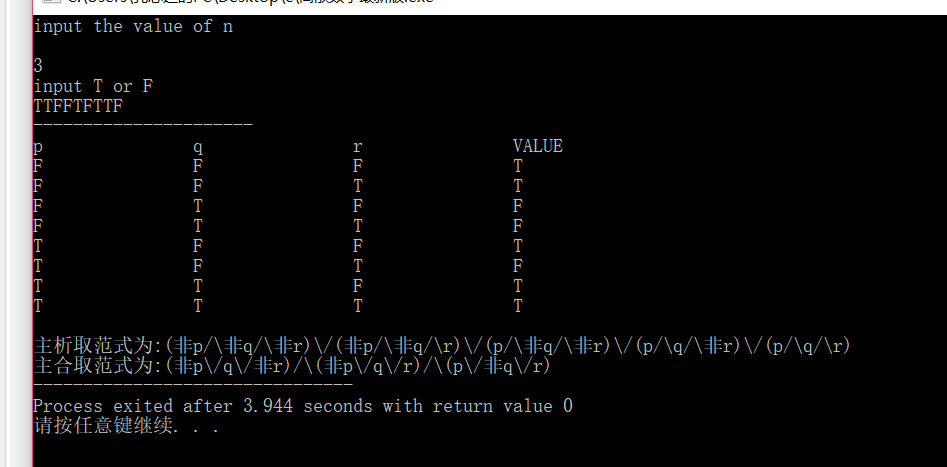展开全文离散数学真值表 C语言编
• 离散数学里面运用C++编写的包括析取合取还有非的运算。离散数学
• 此文件实现了离散中的输出真值表，应用了栈的技术，可以帮助java学习者更好的巩固基础
• 我们介绍了什么是合式公式以及怎样用C语言实现判断机制现在我们来谈谈怎样用C语言去实现合式公式的真值表。 该程序功能就是任意给定一个合式公式我们都能把它的真值表输出出来。步骤1： 引用上一篇的程序，你给我一...

我们介绍了什么是合式公式以及怎样用C语言实现判断机制

现在我们来谈谈怎样用C语言去实现合式公式的真值表。该程序功能就是任意给定一个合式公式我们都能把它的真值表输出出来。

步骤1： 引用上一篇的程序，你给我一个公式首先我得知道它是不是合式公式，这就用到了判断一个公式是否合式公式的方法(详情参照我的上一篇博客)

步骤2： 经过步骤1的检查，我们知道该公式是合式公式，那么就要求真值表了。求真值表本质上也是一个递归的过程，见下图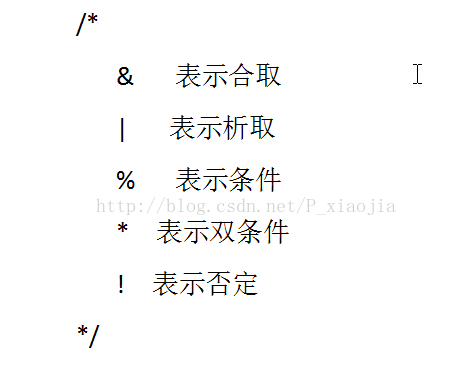步骤3：我们知道了真值表也是个递归的过程，但是合式公式本身只是一串字符无法进行0和1的运算，我们可以让它带上值

利用一下结构体

typedef struct //储存变量带值

{

char ch[Object] ;//储存变量名

bool value[Object] ;//用来放0和1

}Val ;

这样我们就可以把每个变量进行带值运算了 ，当然这里还要把变量从合式公式中抽取出来在放到结构体中。

步骤4：计算变量个数，将变量赋值到结构体数组中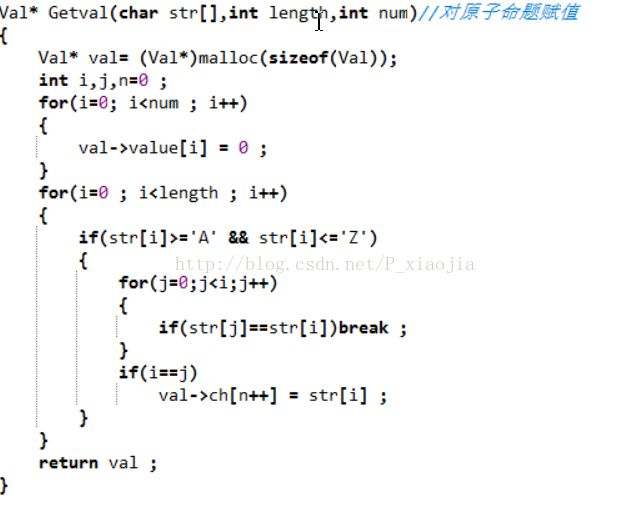步骤5  二级制赋值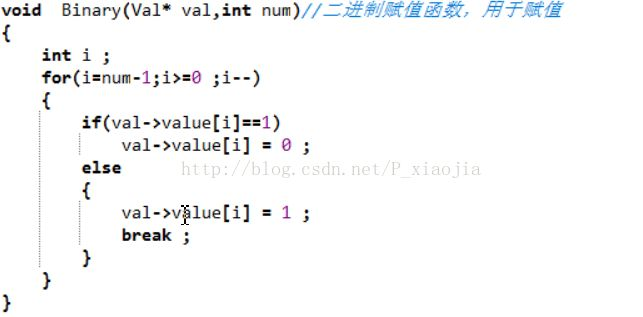步骤6：按分解过程进行计算

最终效果如下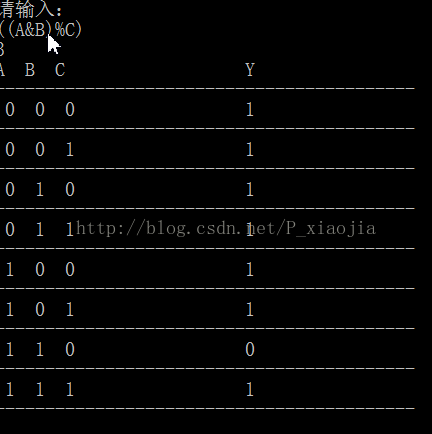展开全文• 离散数学命题公式真值表C或C语言实验报告 离散数学实验报告专业班级：12 级计算机本部一班 姓名：鲍佳珍 学号： 201212201401016 实验成绩：1． 【实验题目】命题逻辑实验二2． 【实验目的】熟悉掌握命题逻辑中...离散数学命题公式真值表C或C语言实验报告

离散数学实验报告专业班级：12 级计算机本部一班 姓名：鲍佳珍 学号： 201212201401016 实验成绩：1． 【实验题目】命题逻辑实验二2． 【实验目的】熟悉掌握命题逻辑中真值表，进一步能用它们来解决实际问题。3． 【实验内容】求任意一个命题公式的真值表4、 【实验要求】C 或 C＋＋语言编程实现5. 【算法描述】1.实验原理真值表:表征逻辑事件输入和输出之间全部可能状态的表格。列出命题公式真假值的表。通常以 1 表示真，0 表示假。命题公式的取值由组成命题公式的命题变元的取值和命题联结词决定，命题联结词的真值表给出了真假值的算法。真值表是在逻辑中使用的一类数学表，用来确定一个表达式是否为真或有效。2.实验过程首先是输入一个合理的式子，生成相应真值表，然后用函数运算，输出结果：要求可生成逻辑非、合取、析取、蕴含、双条件表达式的真值表，例如：输入 !a输出真值表如下：a !a0 11 0输入 avoid yunhan();void xiqu();void shuang();void fei();//声明五个函数int main(){int ch;char s;printf(“欢迎使用命题公式真值表查找软件\n\n“);printf(“1.合取 2.析取 3.蕴含 4.双条件 5.非 0.结束查找\n“);// 软件使用界面的输出scanf(“%d“,while(ch){printf(“\n\n 您能查找真值表的命题公式有以下几种：\n\n“);printf(“1.合取请输入 a//选择输入的公式类型getchar();printf(“请输入您所需要查询的公式：“);scanf(“%s“,s);if(!strcmp(s,“aelse if(!strcmp(s,“!a“))fei();else if(!strcmp(s,“a||b“))xiqu();else if(!strcmp(s,“a-b“))yunhan();else if(!strcmp(s,“ab\n“);printf(“0 1 1\n“);printf(“0 0 1\n“);printf(“1 0 0\n“);printf(“1 1 1\n“);}void fei(){printf(“a !a\n“);printf(“1 0\n“);printf(“0 1\n“);}void shuang(){printf(“a b ab\n“);printf(“0 1 0\n“);printf(“0 0 1\n“);printf(“1 0 0\n“);printf(“1 1 1\n“);} //定义五个函数7． 【实验结果与分析总结(含运行结果截图) 】输入界面输入公式并输出真值表继续查找结束程序

展开全文• 1在命题公式中，对于分量指派真值的各种可能组合，就确定了这个命题公式的各种真值情况，把它汇列成表，就是命题公式的真值表。现举例说明如下：例题1 构造┓p∨q的真值表。解表1-4.1p q ┓p ┓p∨qt t f tt f f ff ...
• } } printf("输入的正整数有%d个，其平均为：%f",nNum,(float)nSum/nNum); return 0; } 3. #include <stdio.h> #include <string.h> int main(void) { int a,nMin,nMax; int i; for(i=0;i;i++){ printf("请...
• 离散数学命题公式求值及真值表题目要求算法思想代码总结 题目要求 给定任意一个命题公式的真值表，并根据真值表求主范式。 算法思想 将逻辑表达式转换为后缀表达式，然后套用逆波兰表达式的求值方法 利用位运算，找...
• 离散数学实验报告专业班级：12级计算机本部一班 姓名：鲍佳珍 学号： 201212201401016 实验成绩：1．【实验题目】命题逻辑实验三2．【实验目的】加深对五个基本联结词(否定、合取、析取、条件、双条件)的理解、...
• 离散数学实验报告专业班级：12 级计算机本部一班学号： 201212201401016姓名：鲍佳珍实验成绩：1．【实验题目】命题逻辑实验二2．【实验目的】熟悉掌握命题逻辑中真值表，进一步能用它们来解决实际问题。3．【实验...
• 离散数学实验二 真值表

千次阅读 2020-06-13 22:08:46
熟悉五个真值表，掌握真值表技术。 二、实验内容 定义1 设命题变元P1、P2、P3、…、Pn是出现在公式G中的所有命题变元，指定P1、P2、P3、…、Pn 的一组真值，则这组真值称为G的一个解释或指派，常记为Ｉ。 定义2 真值...
• 南京邮电大学实验一真值表法求主析取范式和主合取范式代码 离散数学 实 验 一利用真值表法求取主析取范式以及主合取范式的实现 实验名称：利用真值表法求取主析取范式以及主合取范式的实现 实验目的：通过编程实现主...
• * 显示合适公式的真值表 * 提供将一个中缀合适公式的真值表输出到某一PrintStream流中的功能 * 以单个大写字母表示变量（支持26个变量） * 以字符0或者1表示值 * 以 ~ ^ & > - 分别表示 非 析取 合取 条件 双...
• //1.4 写一个程序,包含三个变量和两个逻辑连接词生成真值表. #include<stdio.h> //逻辑联结词的计算 int f( int a,int b,int connection ) { switch( connection ) { //合取 case 0: return a*b;...c语言
• 根据给出的命题数n与对应的真值写出真值表 #include &lt;iostream&gt; #include &lt;math.h&gt; using namespace std; void shuru(char *p,int s);//输入T or F void shuchu(char *p,int s);//...离散数学 编程 真值表
• 已知命题公式A=﹁( p q ) ( (p r) s)，用JAVA或C/C++语言编写程序构造该命题公式的真值表真值表输出样式自己设计(变量值可以不手工输入)，编制程序、画流程图、解释核心程序段、展示结果、心得等，撰写并提交实践...离散数学
• 离散数学实验报告三——真值表与主范式 一、预习内容： 1、真值表：把变量的各种可能取值与想对应的函数值，用表格的形式一一列举出来，这种表格就叫做真值表 2、命题公式的析（合）取范式 ⑴文字：命题变元及其否定...数学
• 代码： #include<stdio.h> #include<math.h>... //brr用来存放真值表 int beg = 80; //字符P对应的ASCII码 int sta1 = 0, sta2 = 0; //sta1表示真值为1的个数，sta2表示真值为0的个数 int mai...